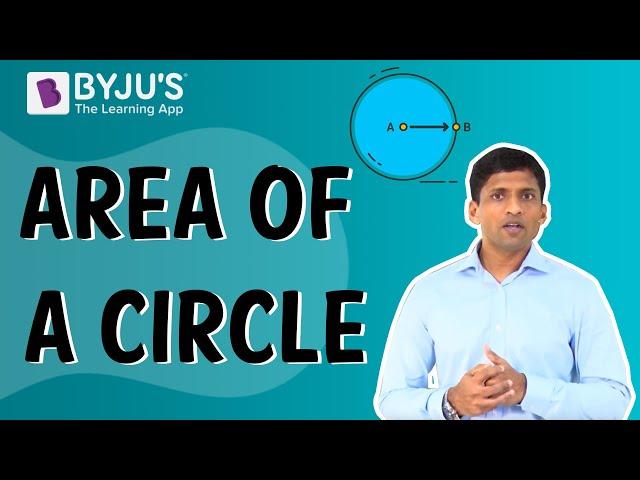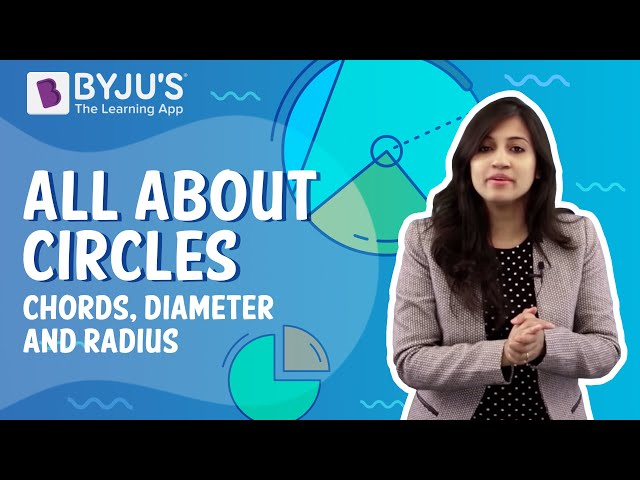# Tangent Of A Circle

Before understanding the concept of the tangent of a circle, let us understand about the circle and how the line intersects the circle in this article.

Also, learn:

## What is a Circle?

A circle is a set of all points in a plane which are equally spaced from a fixed point. The fixed point is called the center of the circle and the distance between any point on the circle and its center is called the radius.

## What is the Tangent of a Circle?

A tangent to a circle is a line which intersects the circle at only one point. The common point between the tangent and the circle are called the point of contact.

Given, a line to a circle could either be intersecting, non-intersecting or just touching the circle or non-touching.

Consider any line AB and a circle. There are 3 possibilities as shown in the below:

(1) Line

$$\begin{array}{l}AB\end{array}$$
intersects the circle at two points
$$\begin{array}{l}P\end{array}$$
and
$$\begin{array}{l}Q\end{array}$$
. Such a line is called secant of the circle.
$$\begin{array}{l}P\end{array}$$
and
$$\begin{array}{l}Q\end{array}$$
are the points on the circle;
$$\begin{array}{l}PQ\end{array}$$
is a chord of the circle.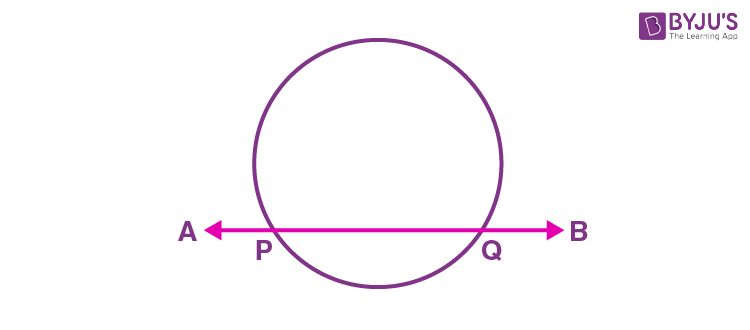(2) Line

$$\begin{array}{l}AB\end{array}$$
touches the circle exactly at one point,
$$\begin{array}{l}P\end{array}$$
. Such a line is called the tangent to the circle.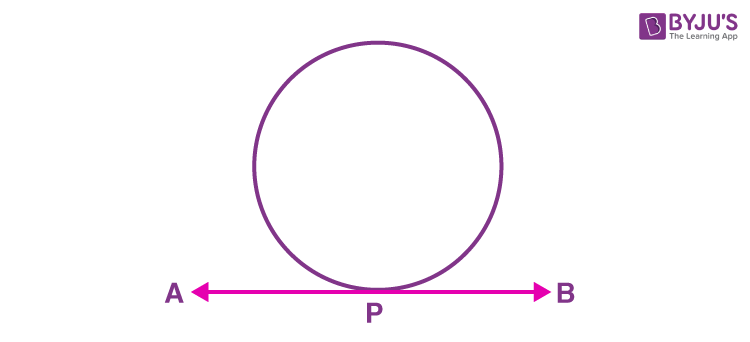(3) Line

$$\begin{array}{l}AB\end{array}$$
does not touch the circle at any point and is referred to as a non-intersecting line.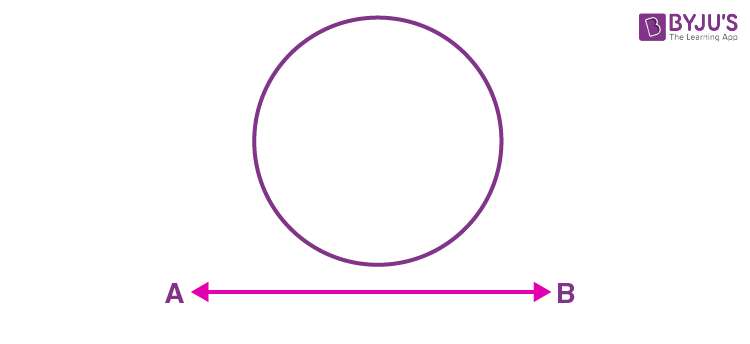### Tangent of a Circle Example

Imagine a bicycle moving on a road. If we look at its wheel, we observe that it touches the road at just one point. The road can be considered as a tangent to the wheel.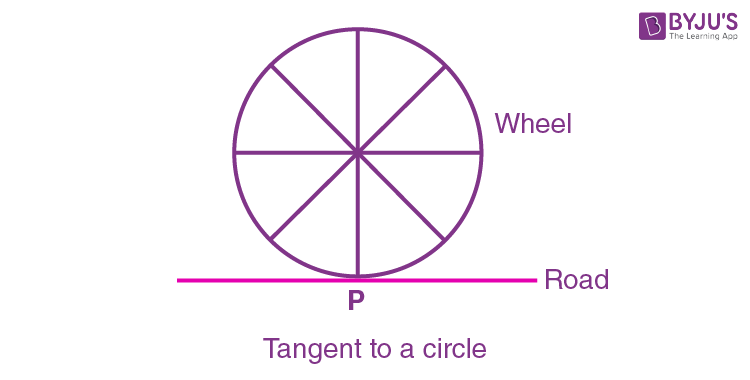It is to be noted that there can one and only one tangent through any given point on the circle.

Any other line through a point on the circle other than the tangent at that point would intersect the circle at two points. This can be easily seen from the following figure.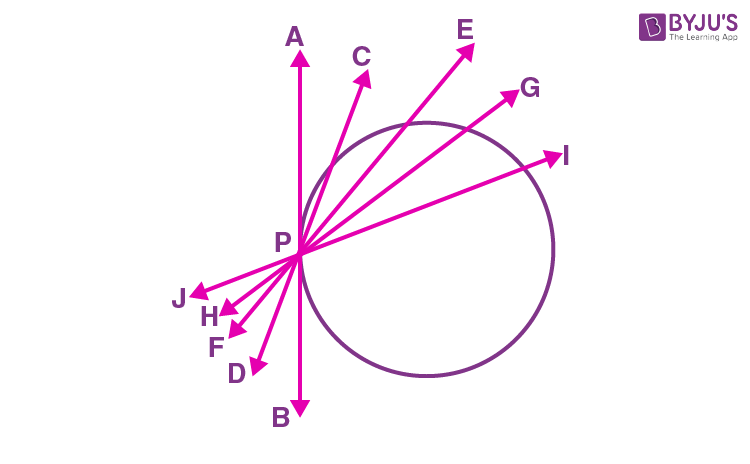$$\begin{array}{l}\overleftrightarrow{AB}, \overleftrightarrow{CD}, \overleftrightarrow{EF}, \overleftrightarrow{GH}, \overleftrightarrow{IJ}\end{array}$$
are a few lines passing through the point
$$\begin{array}{l}P\end{array}$$
, where
$$\begin{array}{l}P\end{array}$$
is a point on the circle. We observe that all the lines except
$$\begin{array}{l}\overleftrightarrow{AB}\end{array}$$
pass through
$$\begin{array}{l}P\end{array}$$
and cut the circle at some other point. Hence, only
$$\begin{array}{l}\overleftrightarrow{AB}\end{array}$$
is a tangent and
$$\begin{array}{l}\overleftrightarrow{CD}, \overleftrightarrow{EF}, \overleftrightarrow{GH}~ and ~\overleftrightarrow{IJ}\end{array}$$
are secants to the circle.

Every secant has a corresponding chord to the circle. Therefore, a tangent can be considered as a special case of secant when the endpoints of its corresponding chord coincide.

## Video Lessons on Circles

### Introduction to Circles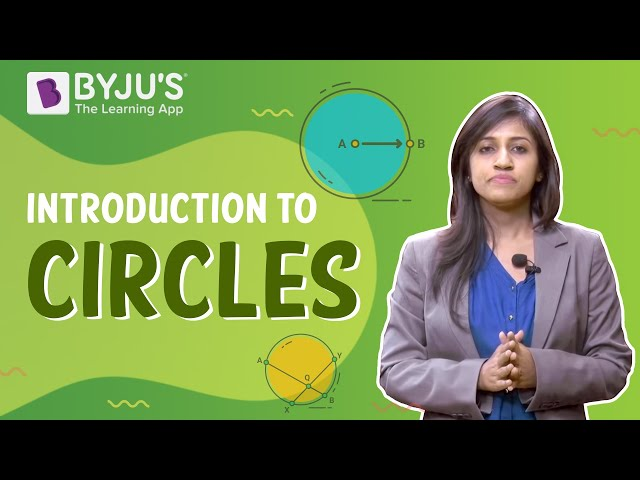### Parts of a Circle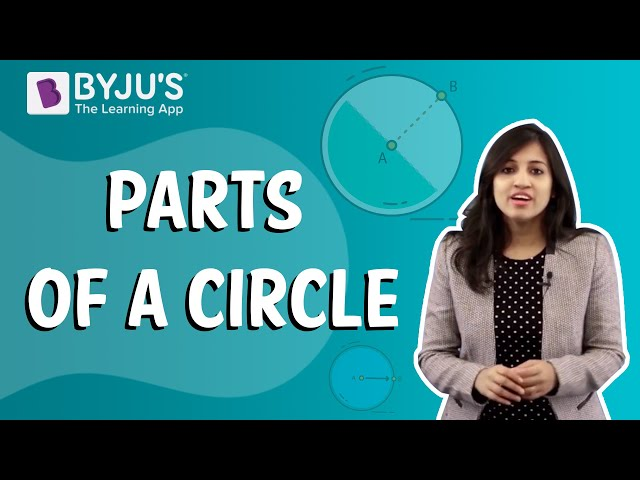### Area of a Circle